## Example Questions

### Example Question #1 : Prisms

The surface area of a rectangular prism is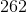, and the lengths of two sides areand. What is the volume of the prism?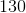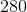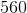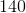Explanation:

The surface area of a rectangular prism with sides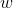, andis given as: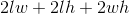Two sides are known; it does not matter how they are designated, but for this problem let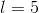and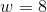, withas the unknown side. This yields equality: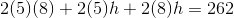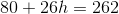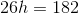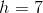Now that the three dimensions are known, it's possible to calculate the volume: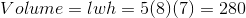### All GRE Math Resources